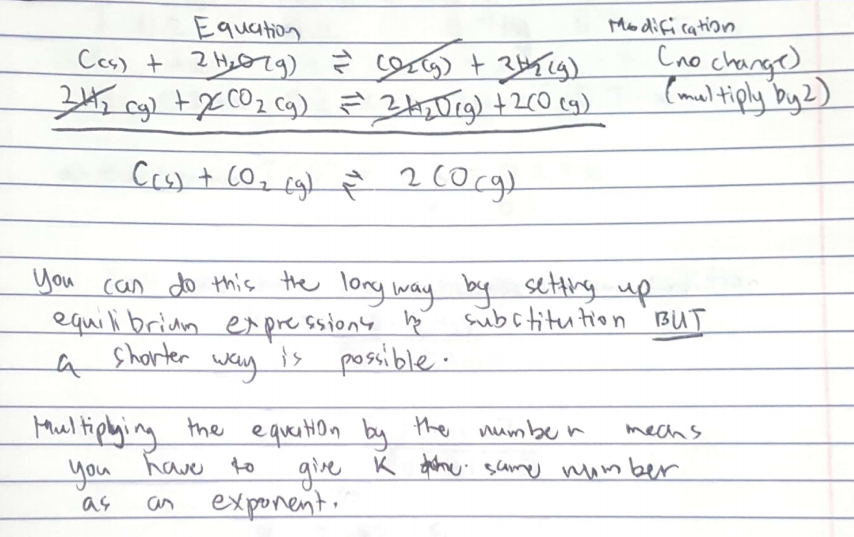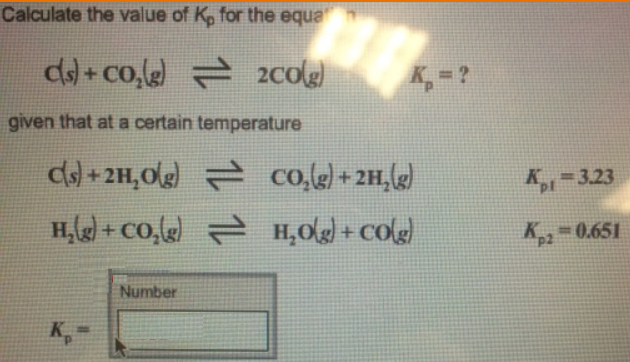# Problem: Calculate the value of Kp for the equation C (s) + CO2 (g) ⇌ 2CO (g) Kp = ? given that at a certain temperature C (s) + 2H2O (g) ⇌ CO2 (g) + 2H2 (g) Kp1 = 3.23 H2 (g) + CO2 (g) ⇌ H2O (g) + CO (g) Kp2 = 0.651

###### FREE Expert Solution93% (291 ratings)###### Problem Details

Calculate the value of Kp for the equation

C (s) + CO2 (g) ⇌ 2CO (g) Kp = ?

given that at a certain temperature

C (s) + 2H2O (g) ⇌ CO2 (g) + 2H2 (g) Kp1 = 3.23

H2 (g) + CO2 (g) ⇌ H2O (g) + CO (g) Kp2 = 0.651